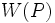# Conjugacy functor that gives a normal subgroup

Jump to: navigation, search
This article defines a property that can be evaluated for a conjugacy functor on a finite group. |View all such properties

## Definition

Suppose$p$ is a prime number and$G$ is a finite group such that$W$ is a conjugacy functor for$G$ for the prime$p$. We say that$W$ is a conjugacy functor that gives a normal subgroup if it satisfies the following equivalent conditions:

1. For every pair of$p$-Sylow subgroups$P,Q$ of$G$,$W(P) = W(Q)$.
2. For every pair of$p$-Sylow subgroups$P,Q$ of$G$,$W(P)$ is a normal subgroup of$Q$.
3. Each of these:
•$W$ is a weakly closed conjugacy functor and there exists a$p$-Sylow subgroup$P$ of$G$ such that$W(P) \le O_p(G)$ where$O_p(G)$ is the p-core of$G$.
•$W$ is a weakly closed conjugacy functor and for every$p$-Sylow subgroup$P$ of$G$,$W(P) \le O_p(G)$ where$O_p(G)$ is the$p$-core of$G$.
4. Each of these:
• There exists a$p$-Sylow subgroup$P$ of$G$ such that$W(P)$ is a normal subgroup of$G$.
• For every$p$-Sylow subgroup$P$ of$G$,$W(P)$ is a normal subgroup of$G$.

### Equivalence of definitions

Further information: equivalence of normality and characteristicity conditions for conjugacy functor

## Relation with other properties

### Weaker properties

Property Meaning Proof of implication Proof of strictness (reverse implication failure) Intermediate notions
conjugacy functor that controls strong fusion element-wise conjugacy in a$p$-Sylow subgroup$P$ is determined in the normalizer of$W(P)$ |FULL LIST, MORE INFO
conjugacy functor that controls fusion subset-wise conjugacy in a$p$-Sylow subgroup$P$ is determined in the normalizer of$W(P)$ Conjugacy functor that controls strong fusion, Conjugacy functor whose normalizer generates whole group with p'-core|FULL LIST, MORE INFO
conjugacy functor whose normalizer generates whole group with p'-core For$P$ a$p$-Sylow subgroup,$O_{p'}(G)N_G(W(P)) = G$ |FULL LIST, MORE INFO
strongly closed conjugacy functor returns a subgroup that is a strongly closed subgroup in the Sylow subgroup relative to the whole group. |FULL LIST, MORE INFO
weakly closed conjugacy functor returns a subgroup that is a weakly closed subgroup in the Sylow subgroup relative to the whole group. Strongly closed conjugacy functor|FULL LIST, MORE INFO